# Merging labels according to centroid-distances#

In this notebook we will merge labels in a label image according to their centroid-distances to each other. Labels close-by will be merged.

import pyclesperanto_prototype as cle


For demonstration purposes, we use a modified version of the labels derived from the blobs example-image. We artificially introduce gaps between them.

image = imread("../../data/blobs.tif")
image[:, 80:150] = 0
image[80:130, 100:] = 0

image = cle.asarray(image)
labels = cle.voronoi_otsu_labeling(image, spot_sigma=4, outline_sigma=3)
labels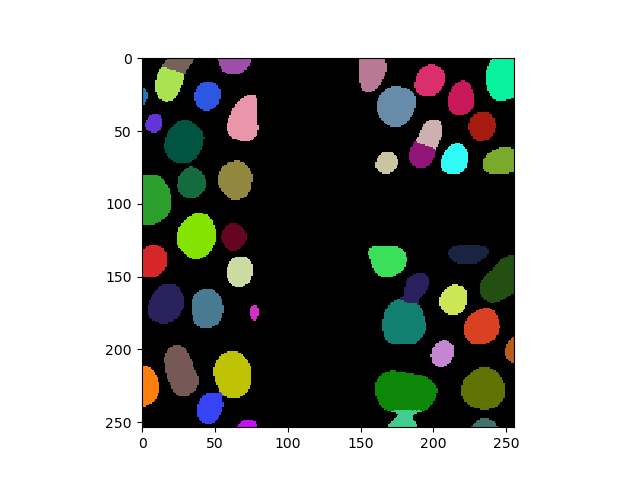cle._ image
 shape (254, 256) dtype uint32 size 254.0 kB min 0.0 max 47.0

From this image, we extract the coordinates of centroids. From these centroids, we can build a distance matrix. In this matrix, the distance from all centroids to all other centroids is computed. The diagonale is zero as it corresponds to the distance of one centroid to itself. Furthermore, the distance to background (first row and first colum) is also zero, as background is not considered for distance computation.

centroids = cle.centroids_of_labels(labels)

distance_matrix = cle.generate_distance_matrix(centroids, centroids)
distance_matrix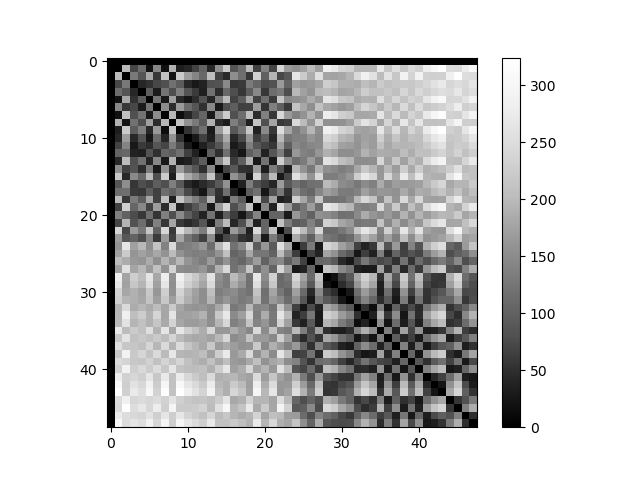cle._ image
 shape (48, 48) dtype float32 size 9.0 kB min 0.0 max 324.1848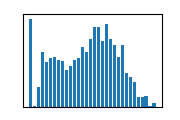We can threshold this distance matrix with a given maximum distance. The result is a binary matrix.

maximum_distance = 40

merge_matrix = distance_matrix <= maximum_distance
merge_matrix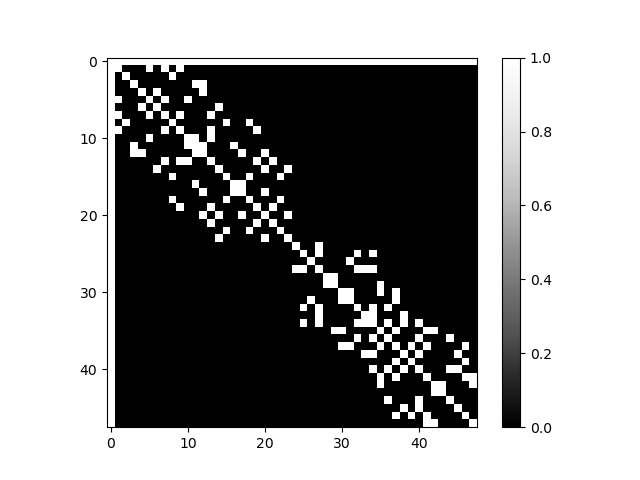cle._ image
 shape (48, 48) dtype uint8 size 2.2 kB min 0.0 max 1.0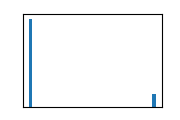If we werged labels with the background, all labels would be merged because all touch the background. In order to prevent this, we set the first row and column to zero.

cle.set_column(merge_matrix, 0, 0)
cle.set_row(merge_matrix, 0, 0)

merge_matrix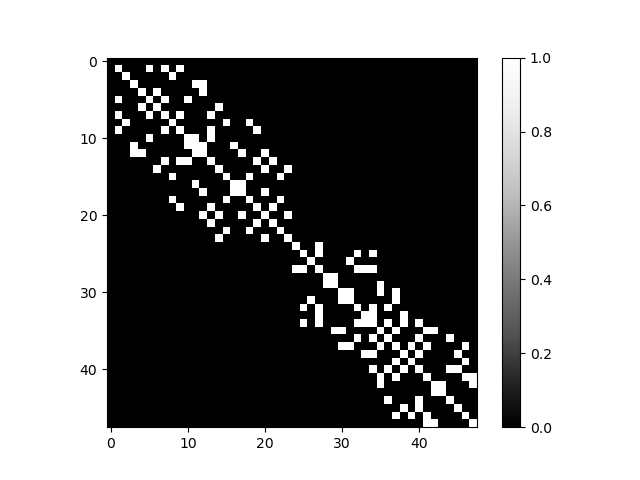cle._ image
 shape (48, 48) dtype uint8 size 2.2 kB min 0.0 max 1.0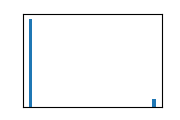Using the binary matrix above we can now merge the labels accordingly.

merged_labels = cle.merge_labels_according_to_touch_matrix(labels, merge_matrix)
merged_labels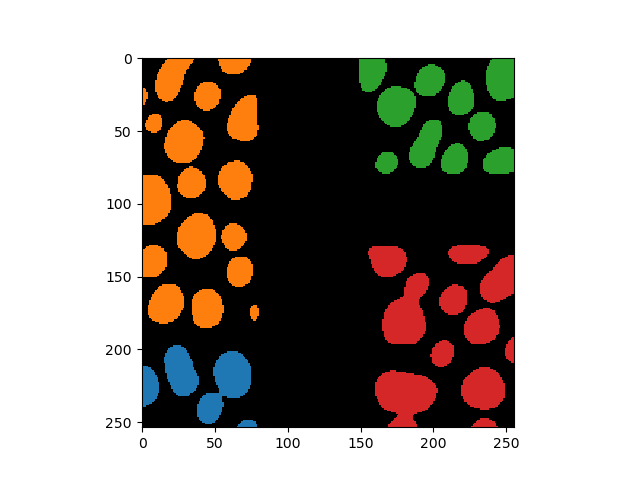cle._ image
 shape (254, 256) dtype uint32 size 254.0 kB min 0.0 max 4.0